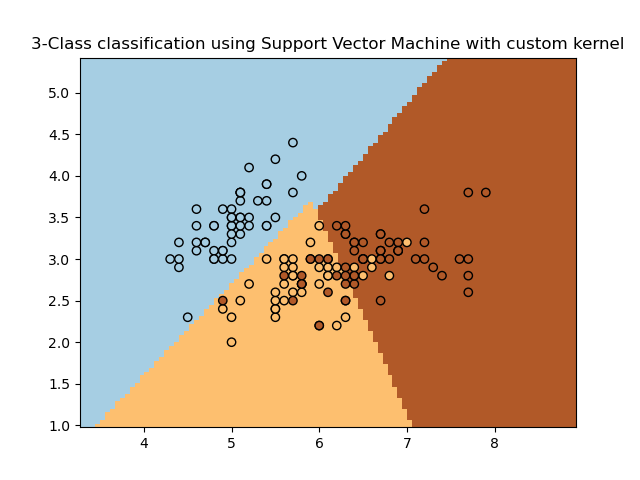# SVM with custom kernel¶

Simple usage of Support Vector Machines to classify a sample. It will plot the decision surface and the support vectors.import numpy as np
import matplotlib.pyplot as plt
from sklearn import svm, datasets
from sklearn.inspection import DecisionBoundaryDisplay

# import some data to play with
X = iris.data[:, :2]  # we only take the first two features. We could
# avoid this ugly slicing by using a two-dim dataset
Y = iris.target

def my_kernel(X, Y):
"""
We create a custom kernel:

(2  0)
k(X, Y) = X  (    ) Y.T
(0  1)
"""
M = np.array([[2, 0], [0, 1.0]])
return np.dot(np.dot(X, M), Y.T)

h = 0.02  # step size in the mesh

# we create an instance of SVM and fit out data.
clf = svm.SVC(kernel=my_kernel)
clf.fit(X, Y)

ax = plt.gca()
DecisionBoundaryDisplay.from_estimator(
clf,
X,
cmap=plt.cm.Paired,
ax=ax,
response_method="predict",
plot_method="pcolormesh",